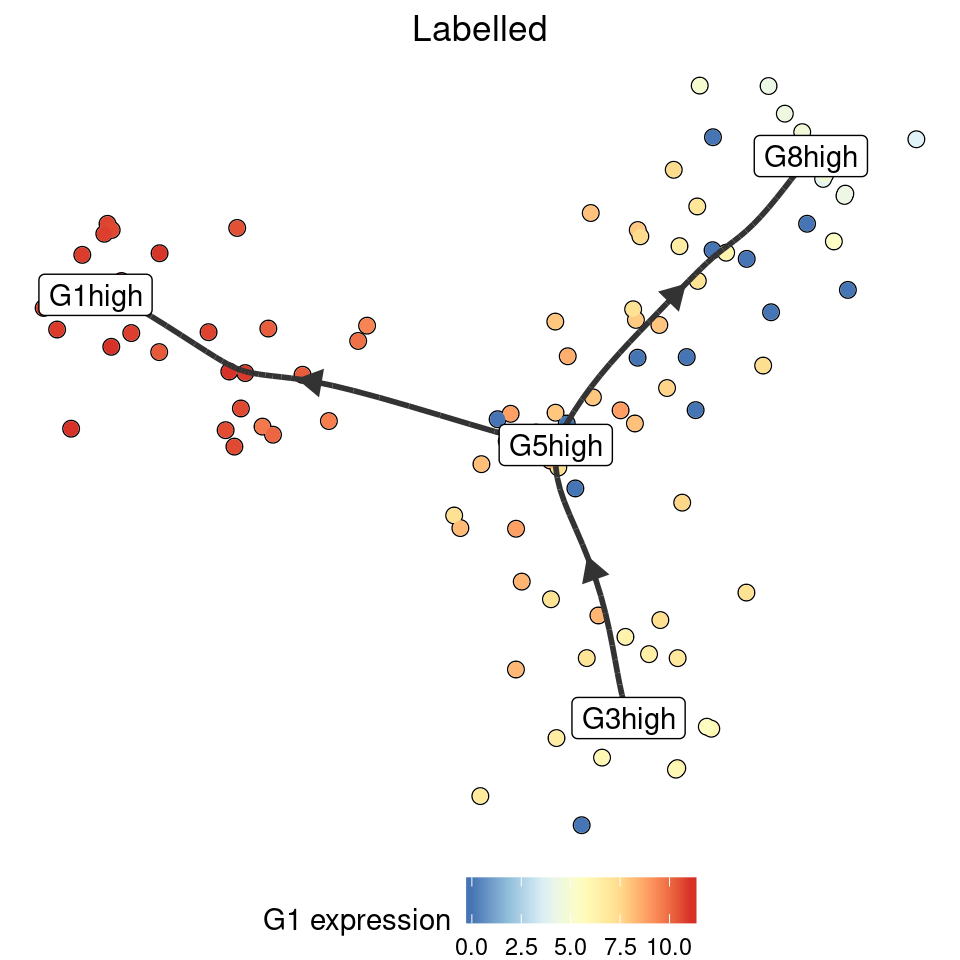``````library(dyno)
library(tidyverse)``````

## Simplifying

Intermediate milestones can be removed by simplyfing the trajectory:

``````model <- dyntoy::generate_dataset(model = dyntoy::model_linear(num_milestones = 10))
simplified <- simplify_trajectory(model)``````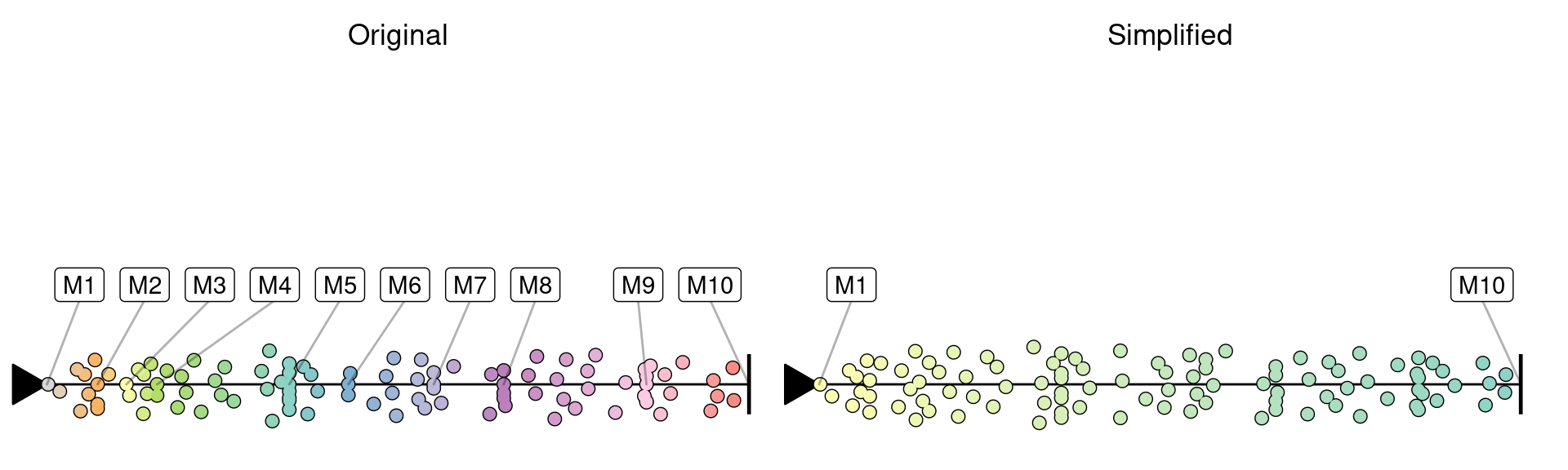## Rooting

TI methods often do not have an idea where the root of a trajectory is. We provide two ways of rooting a trajectory. After rooting, all other edges will point away from the root.

``````set.seed(1)
model <- dyntoy::generate_dataset(model = dyntoy::model_bifurcating())``````

### Manually

If you know the milestone (or cell) that is at the start of the trajectory, you can directly call `add_root`:

``model_rooted <- model %>% add_root(root_milestone_id = "M4")``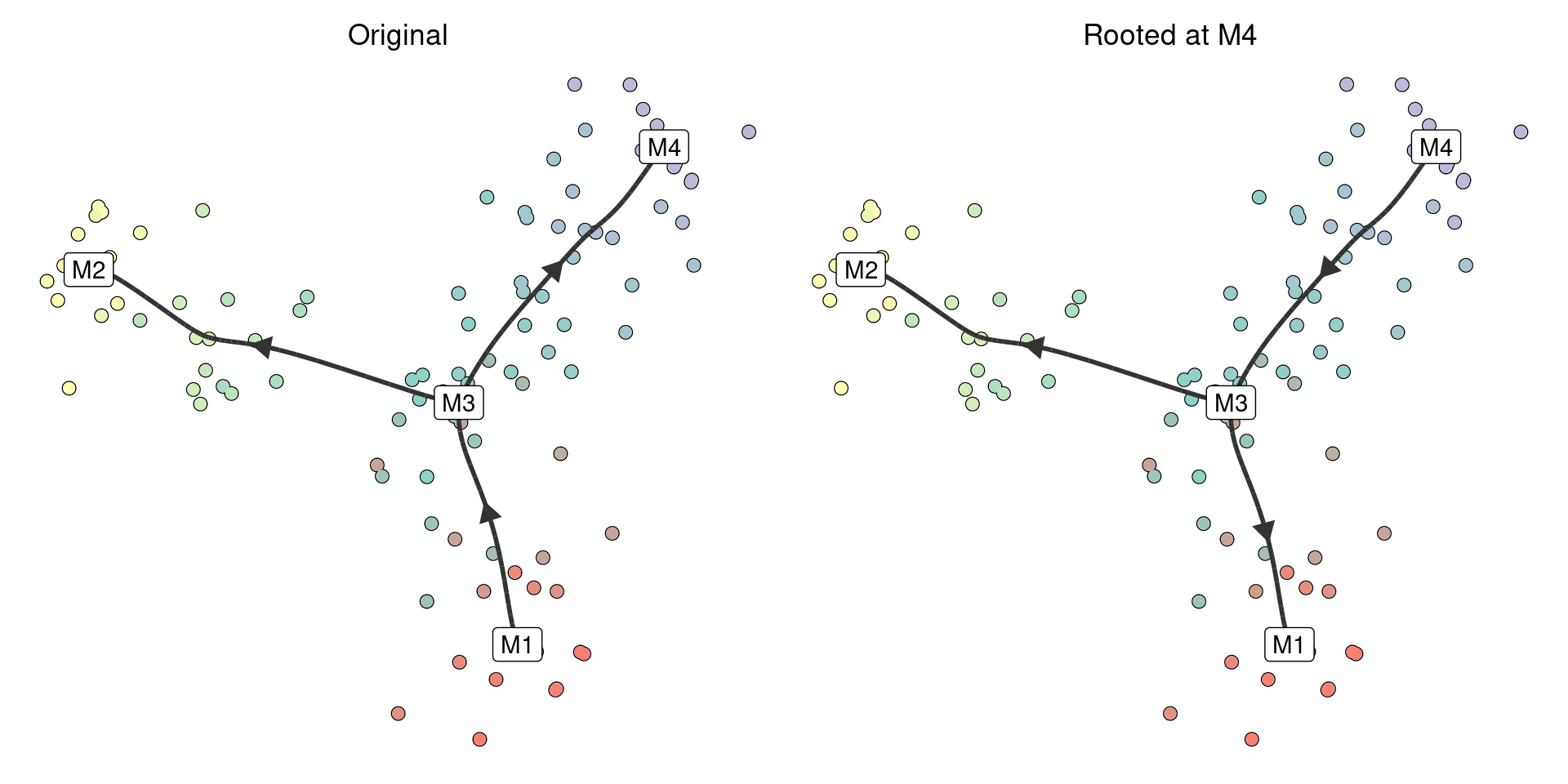### Using marker genes

If you know some marker genes that are highly expressed at the start of the trajectory, rooting can be done implicitely:

``model_rooted <- model %>% add_root_using_expression("G1", expression_source = model)``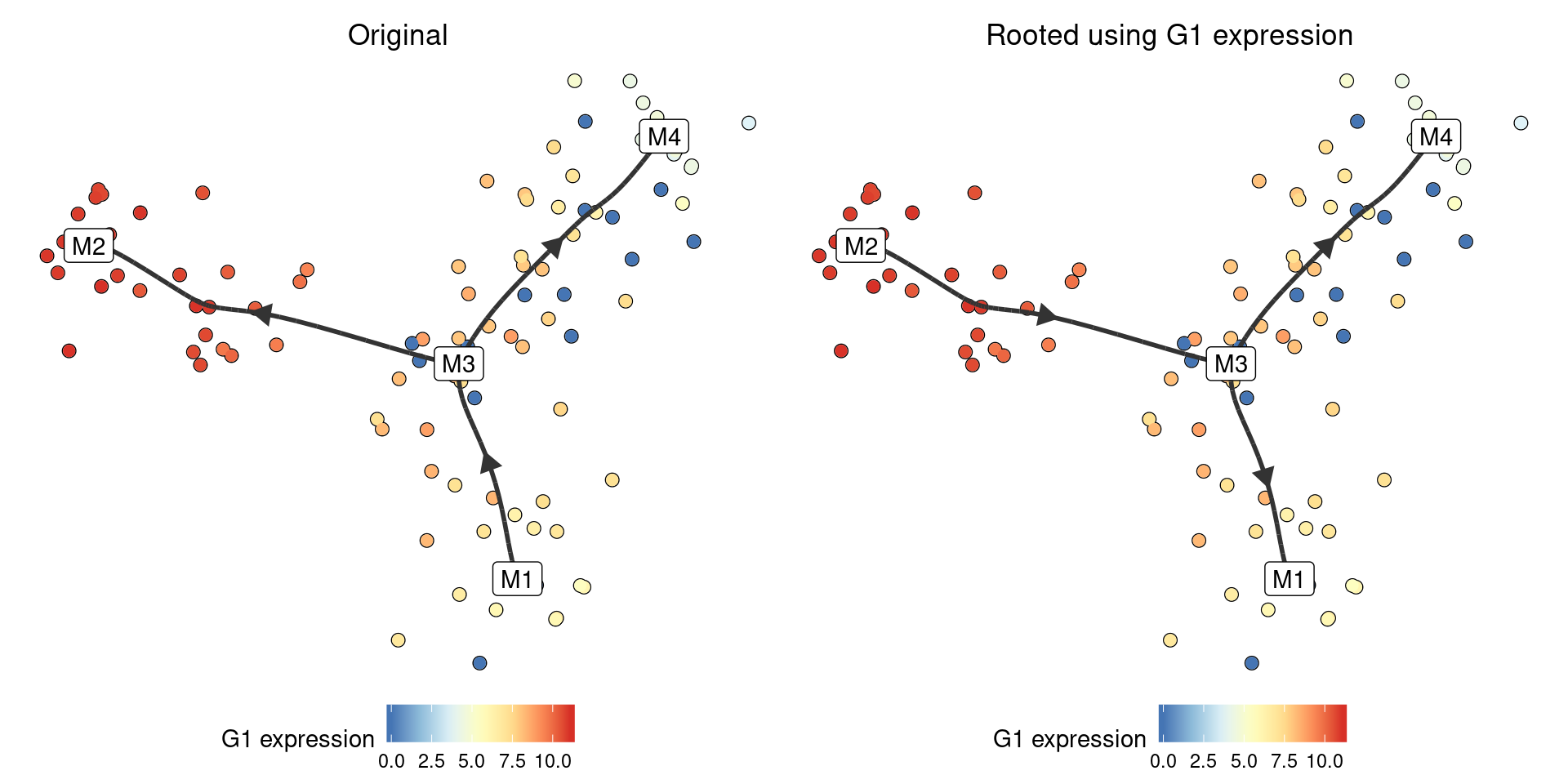## Annotating

Similarly as with rooting, there are also two ways to annotate the milestones within a trajectory:

### Manually

``model_labelled <- model %>% label_milestones(c(M1 = "start", M2 = "end1", M3 = "decision", M4 = "end2"))``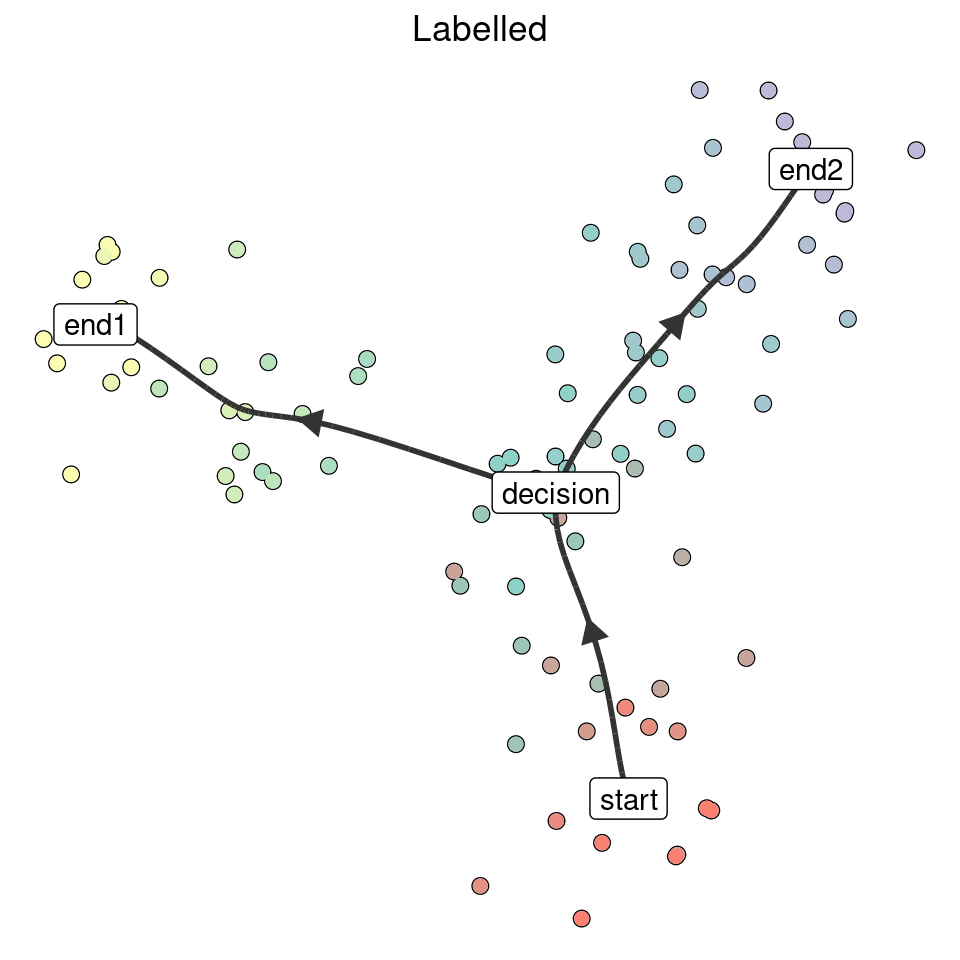### Using marker genes

``````model_labelled <- label_milestones_markers(
model,
markers = list(
G1high = c("G1"),
G5high = c("G5"),
G3high = c("G3"),
G8high = c("G8")
)
)``````